Courses

# Differential Equations - 14

## 20 Questions MCQ Test Topic-wise Tests & Solved Examples for IIT JAM Mathematics | Differential Equations - 14

Description
This mock test of Differential Equations - 14 for Mathematics helps you for every Mathematics entrance exam. This contains 20 Multiple Choice Questions for Mathematics Differential Equations - 14 (mcq) to study with solutions a complete question bank. The solved questions answers in this Differential Equations - 14 quiz give you a good mix of easy questions and tough questions. Mathematics students definitely take this Differential Equations - 14 exercise for a better result in the exam. You can find other Differential Equations - 14 extra questions, long questions & short questions for Mathematics on EduRev as well by searching above.
QUESTION: 1

### The boundary value problem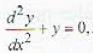x ∈ [0, π], y(0), = 0, y(π) = 0 has

Solution: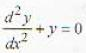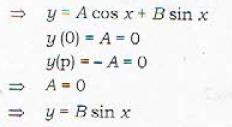QUESTION: 2

Solution:
QUESTION: 3

### A differenlial equation dy/dx = y tanx - 2sin x has an integrating factor

Solution: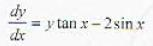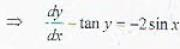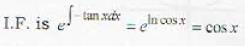QUESTION: 4

Equation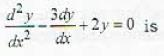Solution:

m2 - 3m + 2 = 0
⇒ m = 1, 2

QUESTION: 5

The general solution of the differential equation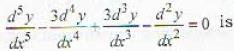Solution: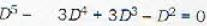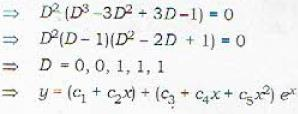QUESTION: 6

The solution of the differential equation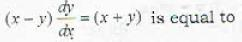where C is a constant.

Solution: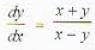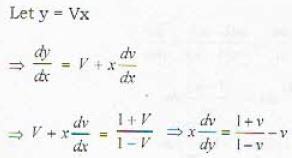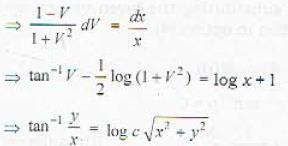QUESTION: 7

The degree of the differential equation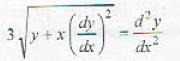Solution: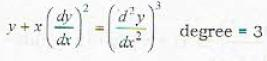QUESTION: 8

The integrating factor of the differential equation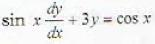is calculated as

Solution: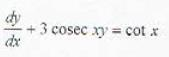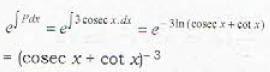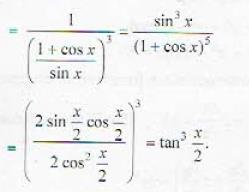QUESTION: 9

The solution of the recurrence relation xn = 2 (xn-1 + xn-2) with x1 = 1 and x2 = 1 - √3 is

Solution: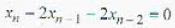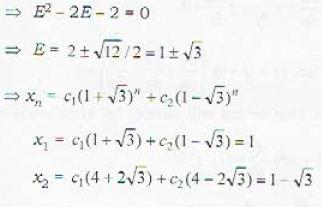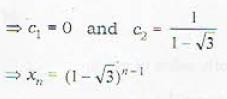QUESTION: 10

Which of the following transformations reduces the given differential equation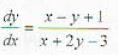into homogeneous one?

Solution: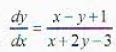If x = X + h and y = Y + k is taken, then h - k + 1 = 0 and h + 2k - 3 =  0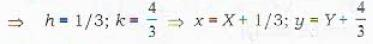QUESTION: 11

The solution of the differential equation y'' + 4y = 0 subject to y(0) = 1, y’ (0) = 2 is

Solution: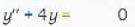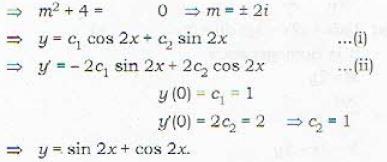QUESTION: 12

General solution of the differential equation x dy = (y+ xe-y/x)dx is given by

Solution: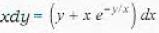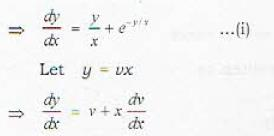So differential equation (1) is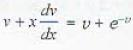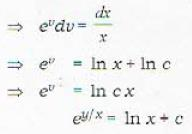QUESTION: 13

A particular solution of the differential equation (D4 + 2D2 - 3) y = ex is

Solution: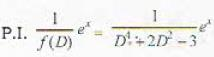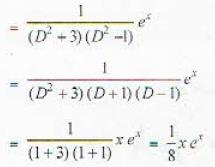QUESTION: 14

A particular solution of the differentia! equation y"'- 3y'' + 3y' - y = ex cos 2x is

Solution:

y''' + 3y" - y = ex cos 2x   .....(i)
It’s particular integral is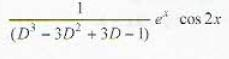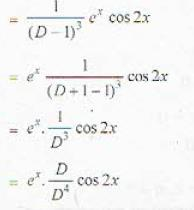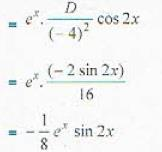QUESTION: 15

If y = In (sin (x + a)) + b, where a and b are constants, is the primitive, then the corresponding lowest order differential equation is

Solution: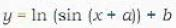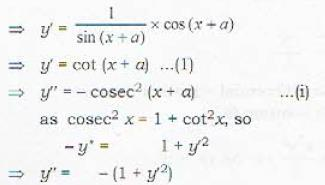QUESTION: 16

Solution of the differential equation xy' + sin 2y = x3 sin2y is

Solution: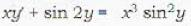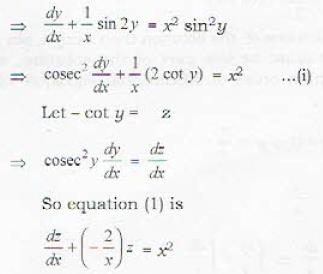which is a linear differential equation, so,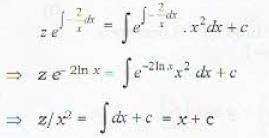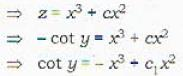QUESTION: 17

The general solution of the differential equation y"' +  y" - y' - y = 0 is

Solution: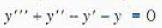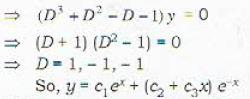QUESTION: 18

The solution of the differential equation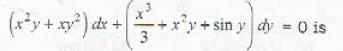Solution:

For the given differential equation
M = x2y+ xy2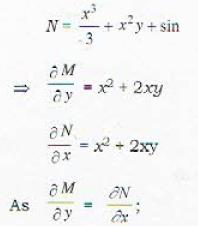so the differential equation is exact so, its solution is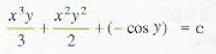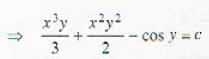QUESTION: 19

Let y[x) = x sin x be one of the solution of an nth order linear differential equation with constant coefficients. Then the minimum value of n is

Solution:

x sin x is one of the solution then x cos x, sin x, cos x must be the part of the solution, so minimum order of diffeerenlial equation will be 4.

QUESTION: 20

The solution of the initial value problem xy’ - y = 0 with y(1) = 1 is

Solution: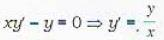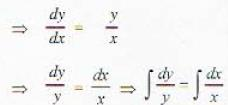In y = In x + In c
y = cx
Al x = 1; y = 1, so eq. (1) becomes
1 = C. 1 ⇒ C= 1
so y(x) = x.